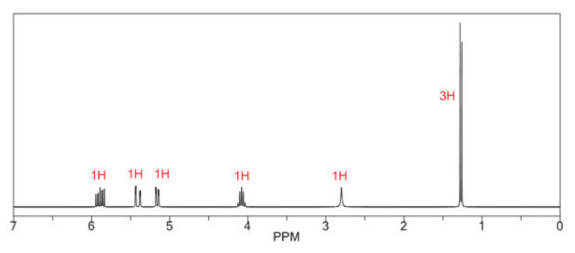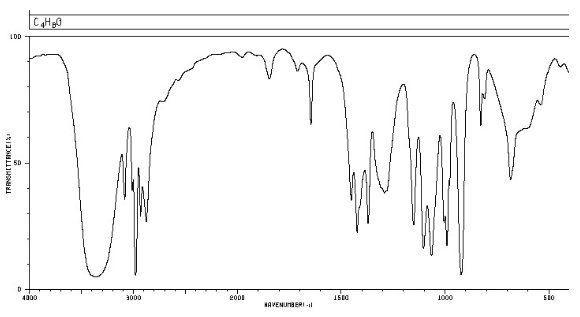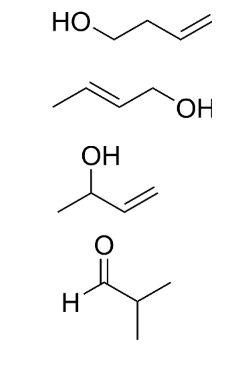# Problem: Select the molecule (C4H8O) that would give the 1H NMR and IR spectra shown below.

🤓 Based on our data, we think this question is relevant for Professor Sacui's class at MEMPHIS.

###### FREE Expert Solution

Given: C4H8O

IHD:

$\mathbf{IHD}\mathbf{=}\frac{\mathbf{2}\mathbf{\left(}\mathbf{4}\mathbf{\right)}\mathbf{+}\mathbf{2}\mathbf{-}\mathbf{\left[}\mathbf{8}\mathbf{\right]}}{\mathbf{2}}$= 1

IHD = 1 means ring or double bond###### Problem Details

Select the molecule (C4H8O) that would give the 1H NMR and IR spectra shown below.